Test: Electrical Machines- 6

# Test: Electrical Machines- 6 - Electrical Engineering (EE)

Test Description

## 10 Questions MCQ Test GATE Electrical Engineering (EE) 2024 Mock Test Series - Test: Electrical Machines- 6

Test: Electrical Machines- 6 for Electrical Engineering (EE) 2023 is part of GATE Electrical Engineering (EE) 2024 Mock Test Series preparation. The Test: Electrical Machines- 6 questions and answers have been prepared according to the Electrical Engineering (EE) exam syllabus.The Test: Electrical Machines- 6 MCQs are made for Electrical Engineering (EE) 2023 Exam. Find important definitions, questions, notes, meanings, examples, exercises, MCQs and online tests for Test: Electrical Machines- 6 below.
Solutions of Test: Electrical Machines- 6 questions in English are available as part of our GATE Electrical Engineering (EE) 2024 Mock Test Series for Electrical Engineering (EE) & Test: Electrical Machines- 6 solutions in Hindi for GATE Electrical Engineering (EE) 2024 Mock Test Series course. Download more important topics, notes, lectures and mock test series for Electrical Engineering (EE) Exam by signing up for free. Attempt Test: Electrical Machines- 6 | 10 questions in 30 minutes | Mock test for Electrical Engineering (EE) preparation | Free important questions MCQ to study GATE Electrical Engineering (EE) 2024 Mock Test Series for Electrical Engineering (EE) Exam | Download free PDF with solutions
 1 Crore+ students have signed up on EduRev. Have you?
Test: Electrical Machines- 6 - Question 1

### A 2 pole, 50 Hz single phase induction motor running at 2600 rpm has an effective rotor resistance and reactance each of 0.5 Ω. The stator impedance and magnetizing current are to be neglected. The frequencies of rotor current in forward branch and in backward branch are to be respectively

Detailed Solution for Test: Electrical Machines- 6 - Question 1

Synchronous speed,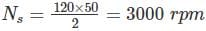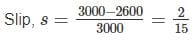Frequency of forward component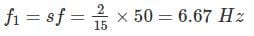Frequency of backward component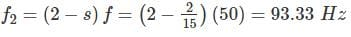*Answer can only contain numeric values
Test: Electrical Machines- 6 - Question 2

### A 250 V, 700 W, 50 Hz, 6 pole single phase induction motor is rotating is clockwise direction with a speed of 960 rpm. The rotor resistance at standstill is 10 Ω. What is the effective rotor resistance is the backward branch of the equivalent circuit in ohms?

Detailed Solution for Test: Electrical Machines- 6 - Question 2

Synchronous speed, NS = 120f/p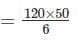= 1000 rpm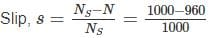= 0.04
Resistance of backward field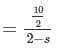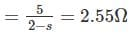Test: Electrical Machines- 6 - Question 3

### The direction of rotation of a single-phase capacitor run induction motor is reversed by

Detailed Solution for Test: Electrical Machines- 6 - Question 3

By interchanging the terminals of the auxiliary winding, the direction of rotation of the rotating magnetic field get reverse and hence rotor.

Test: Electrical Machines- 6 - Question 4

A 50 Hz, 6 pole, single phase induction motor is rotating in the clockwise direction at a speed of 940 rpm. The slip of motor in the direction of rotation and opposite direction of motor will be respectively.

Detailed Solution for Test: Electrical Machines- 6 - Question 4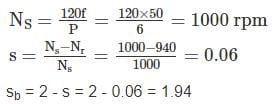Test: Electrical Machines- 6 - Question 5

The starting current and torque of a three phase induction motor on direct line starting is 45 A and 600 Nm respectively. What are the corresponding values with star delta starter.

Detailed Solution for Test: Electrical Machines- 6 - Question 5

Starting current with star delta starter
1/3 of direct on line starting current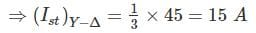Starting torque (Tst) with Y - ∆ starter = 1/3 of DOL starting torque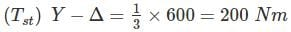*Answer can only contain numeric values
Test: Electrical Machines- 6 - Question 6

The value of the starting capacitor required to produce 90° phase difference between the currents in the main and auxiliary windings will be – (in μF)

Detailed Solution for Test: Electrical Machines- 6 - Question 6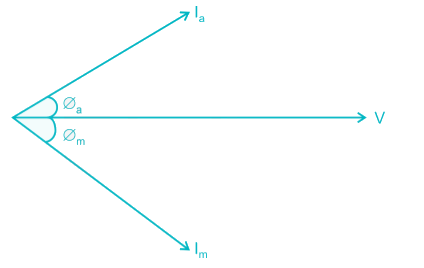Given that ∅a + ∅m = 90°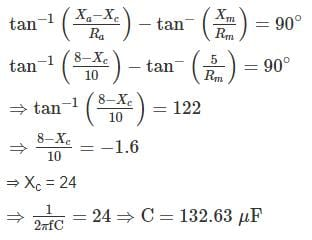*Answer can only contain numeric values
Test: Electrical Machines- 6 - Question 7

An 8 pole 40 kW, 125 V, 50 Hz single phase motor is running at speed at 720 rpm with forward field torque of 200 N-m end backward field torque of 40 N-m. If friction and winding losses are 20 w, what is the mechanical power output in watts?

Detailed Solution for Test: Electrical Machines- 6 - Question 7

Synchronous watts = Net torque
= Forward torque – Backward torque
= 200 – 40
= 160 N-m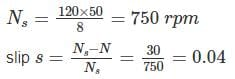watts converted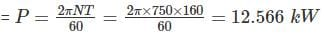Watts converted = 12.566 (1 - s)
= 12.566 (1 – 0.04)
= 12063.3 W
Mechanical power output = 12063.3 – 20
= 12043.3 W

Test: Electrical Machines- 6 - Question 8

A 6 pole, 350 W, 110 V, 50 Hz single phase motor is running at 960 rpm with forward field torque of 220 N-m and backward field torque of 30 N-m. If friction and winding losses are 10 W, What is the mechanical power output?

Detailed Solution for Test: Electrical Machines- 6 - Question 8

Synchronous watt = net torque
= Forward torque – Backward torque
= 220 – 30
= 190 N – m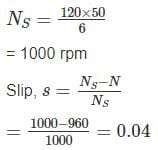Watts converted = 190 (1 – 0.04)
= 182.4 W
Mechanical power output = 182.4 – 10
= 172.4 W

Test: Electrical Machines- 6 - Question 9

Determine the current drawn from the main as a fraction of full load current of an auto transformer starter for an induction motor required to start the motor with 50 percent of full-load torque. The short circuit current of the motor is 6 times the full load current and full load slip is 0.05.

Detailed Solution for Test: Electrical Machines- 6 - Question 9

We know that,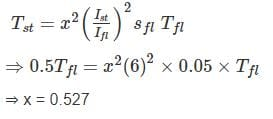Current drawn from the supply =  x2 Ist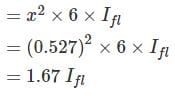*Answer can only contain numeric values
Test: Electrical Machines- 6 - Question 10

A induction motor is started in first case by autotransformer with 3/4th tapping and is second case by a star delta starter. What is the ratio of supply current at starting of first case to second one upto two decimal places

Detailed Solution for Test: Electrical Machines- 6 - Question 10

In the case of autotransformer starter, per phase starting current, Ist = x2Isc
= (0.75)2Isc
= 0.5625 Isc
For star delta starter,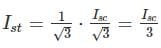= 0.33 Isc
The ratio of first case to second is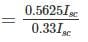= 1.6876
≈ 1.69

## GATE Electrical Engineering (EE) 2024 Mock Test Series

23 docs|285 tests
Information about Test: Electrical Machines- 6 Page
In this test you can find the Exam questions for Test: Electrical Machines- 6 solved & explained in the simplest way possible. Besides giving Questions and answers for Test: Electrical Machines- 6, EduRev gives you an ample number of Online tests for practice

## GATE Electrical Engineering (EE) 2024 Mock Test Series

23 docs|285 tests